# Vector Combine Node

Access: Nodes > Misc > Vector Combine

The Vector Combine node combines components of four input vectors into a single output vector. This node has been designed to be very flexible and allows you to have complete control over the individual components of a vector. The Vector Combine node can be used to extract or make adjustments to the R, G, B and A components separately and then reconstruct them to output a new vector.

Vector Combine is often used after a Vector Split node to stitch a vector back together after it has been separated.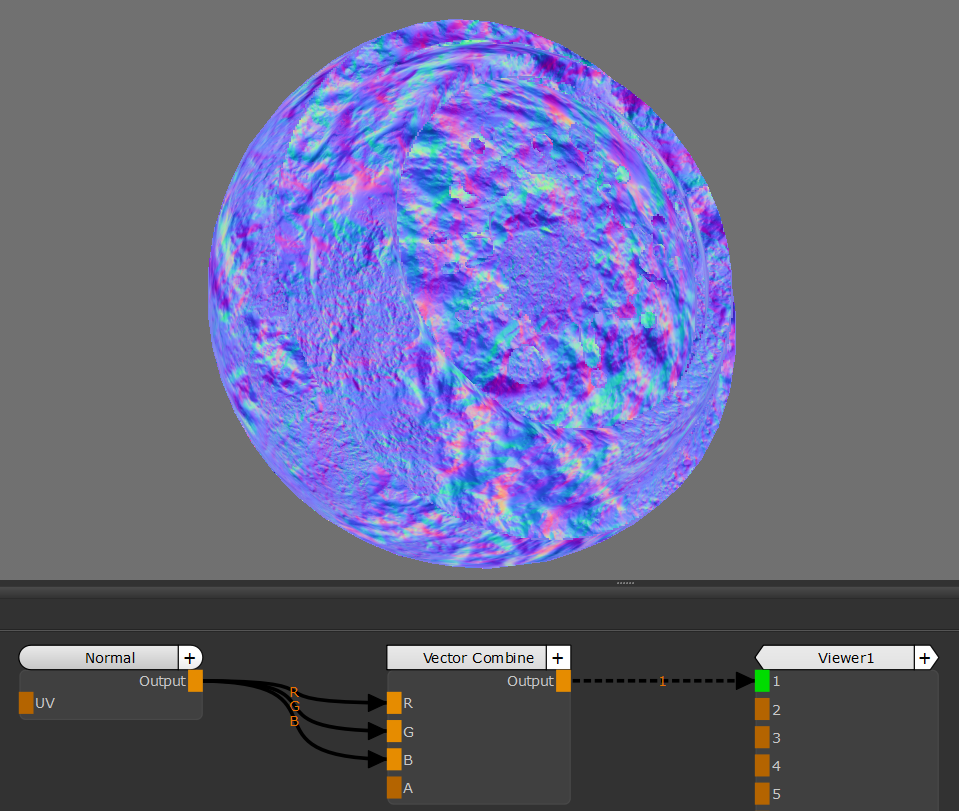R, G and B input taking the respective components from the
vector input resulting in an unchanged vector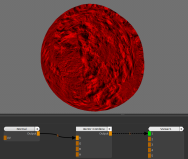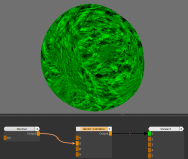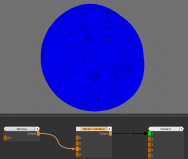R input taking the red component from the input vector G input taking the green component from the input vector B input taking the blue component from the input vector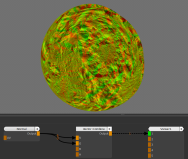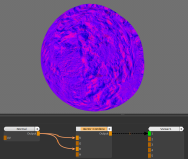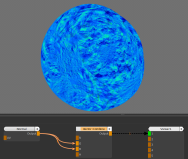R and G inputs taking the respective components from the input vector R and B inputs taking the respective components from the input vector G and B inputs taking the respective components from the input vector

# Vector Combine Node Inputs

 R The vector to use as the input for the red component. If nothing is input, this will default to the red component (the first field) of the Default Inputs attribute in the Node Properties. G The vector to use as the input for the green component. If nothing is input, this will default to the green component (the second field) of the Default Inputs attribute in the Node Properties. B The vector to use as the input for the blue component. If nothing is input, this will default to the blue component (the third field) of the Default Inputs attribute in the Node Properties. A The vector to use as the input for the alpha component. If nothing is input, this will default to the alpha component (the forth field) of the Default Inputs attribute in the Node Properties.

# Vector Combine Node Outputs

 Output This outputs a vector comprised of the chosen components of the input vectors.

# Vector Combine Node Properties

Default Inputs

text field, slider

This defines the default component values to use if the node has nothing connected to their respective input ports.

Note:  Click the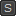button to open the text fields for the individual vector components to use as the Default Inputs.

Input R Component

dropdown

Select the component of the input R to use as the red component for the output vector.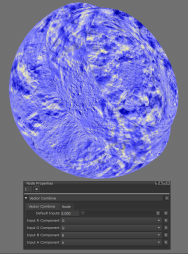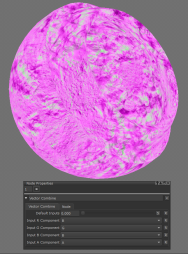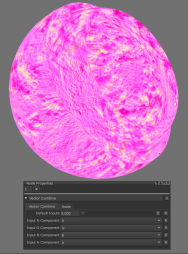G input used as R Component B input used as R Component A input used as R Component

Input G Component

dropdown

Select the component of the input G to use as the green component for the output vector.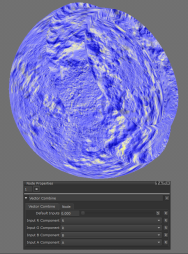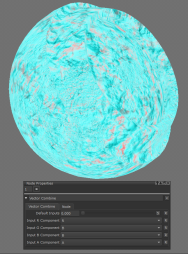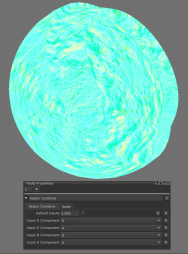R input used as G Component B input used as G Component A input used as G Component

Input B Component

dropdown

Select the component of the input B to use as the blue component for the output vector.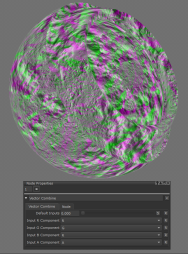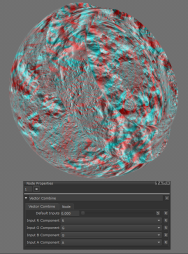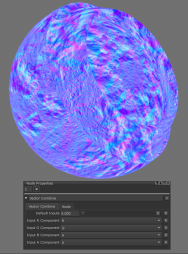R input used as B Component G input used as B Component A input used as B Component

Input A Component

dropdown

Select the component of the input A to use as the alpha component for the output vector.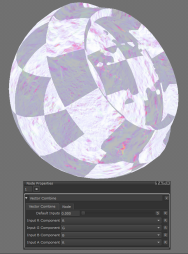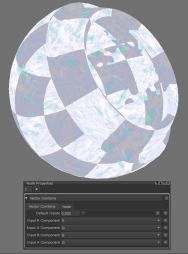R input used as A Component G input used as A Component B input used as A Component

# Workflow Example

Vector Combine is closely linked to the Vector Split node and the two are usually used together. One common workflow is to use Vector Split to separate out individual components, make adjustments to one or more, and then feed the modified component back using Vector Combine.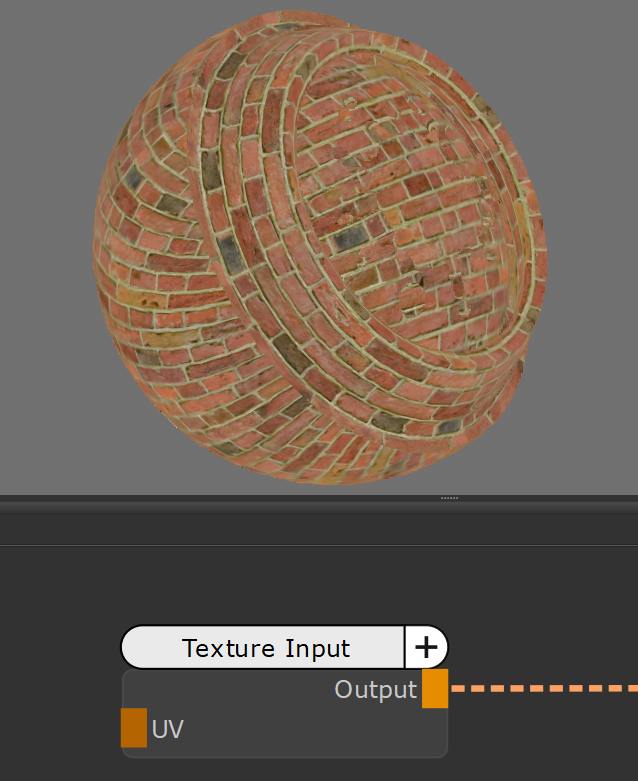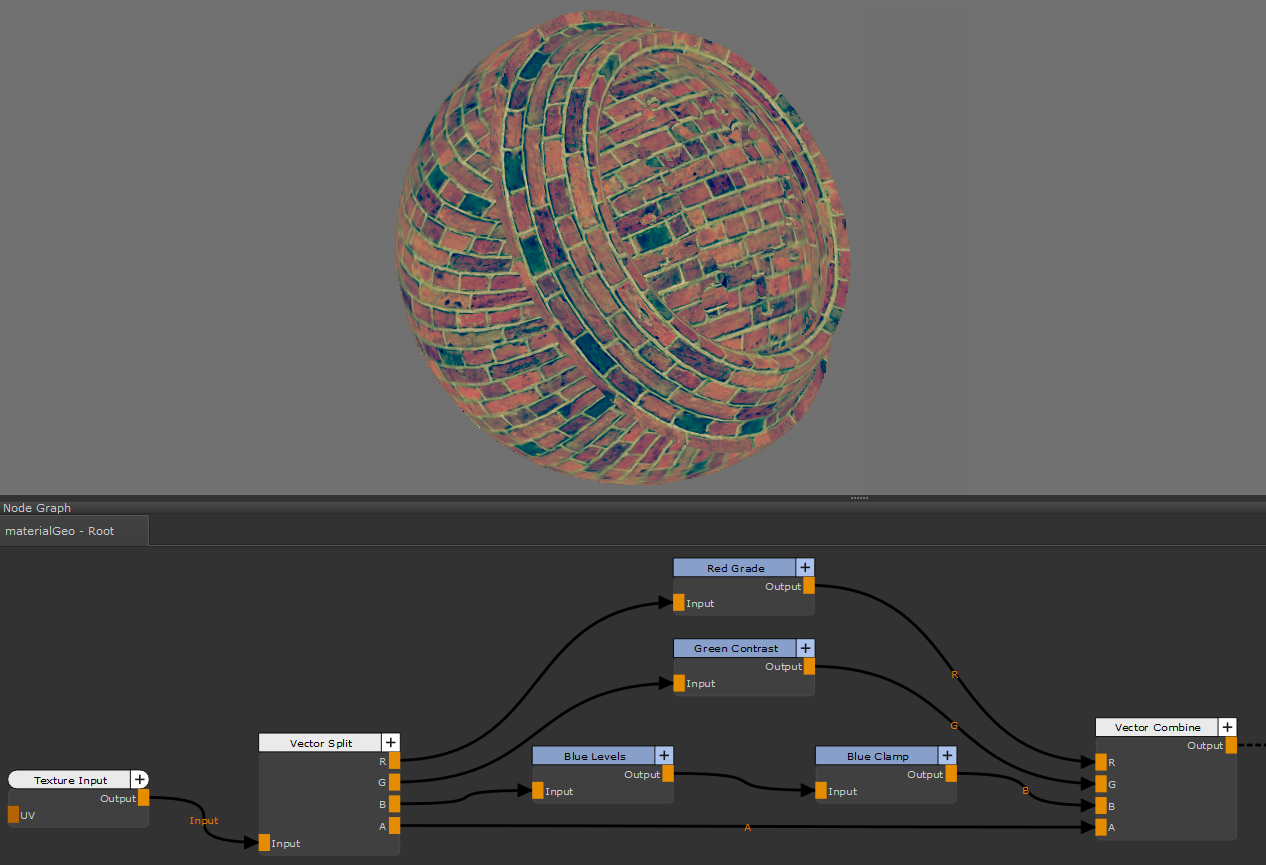Texture Input Texture Output after Adjustments

This use of the Vector Split and Vector Combine nodes can be especially useful when working with textures for video games. These textures are often packed which means multiple channels, such as Roughness, AO and Specular, are stored in a single channel in an image. The Vector Split node allows you to separate these components to use in Mari and the Vector Combine node can then be used to put them back together to export to a game engine.